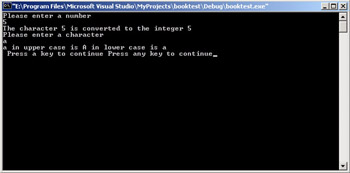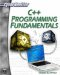# Built-in Functions

In addition to the various functions you can create, C++ includes some useful functions you can use. Actually the term built-in is not exactly accurate, but it is close enough. These are functions that can be included in your program and then use. In fact, there are quite a few such functions. This book will show you some of the most commonly used functions and also show you some examples of how to use these functions. A few of the more commonly used functions are summarized in Table 4.1.

Table 4.1: C++ Functions

Function

Purpose

void *memcpy(*destination, *source, size);

This function copies whatever is in the source (often an array or structure [see Chapter 8]) to the destination. The size tells you how much to copy. To use this function you must either include the memory or string header files.

void * memset(*destination, replacement, size)

This function sets the destination to the character shown in replacement. This is often used to set an array to all zeros. To use this function you must either include the memory or string header files.

char* itoa( int value, char* buffer, int radix ) Note: This one is NOT ANSI Standard C++ but is quite commonly used and supported by most C++ compilers.

This takes an integer and returns the character equivalent. The radix is the base of the integer you are converting it from (base 2, base 10, etc.).

int atoi( const char *string);

This function takes a character and returns the integer equivalent.

int tolower( int c );

Converts the character to lowercase. You must include the string header file for this function.

int toupper( int c );

Converts the character to uppercase. You must include the string header file for this function.

 Hint! memcpy and memset are frequently used with structures, which will be described in detail in Chapter 8. They can, however, also be used with arrays, which were introduced in Chapter 3.

The memcpy function shown in Table 4.1 is used to copy complex data types, such as arrays and structures, from one location in memory to another. You cannot simply copy one array to another as you would copy a standard variable. The following is an example.

`int x,y; x = 4 y = x `

This works fine, and the contents of x are copied to y. However, this does not work with arrays.

`int x = {2,4,1,6,6,9,0,11,1,2}; int y; y = x;`

This will not work. It will generate a compiler error. So how do you copy one array to another? That is where memcpy comes in. To use memcpy, you must first include the memory header file.

`#include <iostream> #include <memory> using namespace std; int main() {      int x = {1,2,3,4,5,6,7,8,9,0};      int y;      memcpy(y,x,10);  return 0; }`

The function copies items from the second parameter to the first parameter, from the source to the destination. It copies the number of bytes you indicate in the third parameter, the size parameter. You will find these functions, and others, used throughout this text, and in many other C++ books. Let’s take a look at an example that uses a few of these.

### Example 4.7

Step 1: Type the following code into your favorite text editor.

`#include <iostream>  using namespace std; int main() {  char a,u,l;  int x;  cout << "Please enter a number \n";  cin >> a;  x = atoi(&a);  cout << "The character " << a << " is converted to     the integer " << x << "\n"; cout << "Please enter a character \n";  cin >> a;  u = toupper(a);  l = tolower(a);    cout << a << " in upper case is " << u << " in lower case is " << l;  cout << "\n Press a key to continue ";  return 0; }`

Step 2: Compile the code.

Step 3: Run the code. You should see something similar to what is shown in Figure 4.7.Figure 4.7: Assorted functions.

 Hint! The & operator is used with the itoa function. This is the addressof operator and means that we are passing the address of the variable rather than the actual value contained in the variable.

The previous example illustrated the most commonly encountered of C++’s built-in functions. These functions will be quite useful to you as you continue through this book. Several of them will be used in later chapters. It would be well worth your time to ensure that you are well-acquainted with each of these functions.C++ Programming Fundamentals (Cyberrookies)
ISBN: 1584502371
EAN: 2147483647
Year: 2005
Pages: 197
Authors: Chuck Easttom